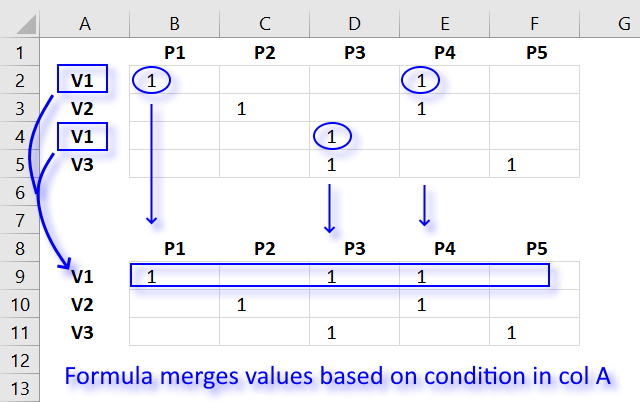Author: Oscar Cronquist Article last updated on November 20, 2019This article explains how to merge values row by row based on a condition in column A using an array formula.

Oscar,

I'm hoping you can help. I am trying to group a number of rows together by the first column, providing a union of the column values. In the example below, we are looking to Vendors V1-V3 to sell us some subset of products P1-P5.

; P1 ; P2 ; P3 ; P4 ; P5
V1 ; 1 ; ; ; 1 ;
V2 ; ; 1 ; ; 1 ;
V1 ; ; ; 1 ; ;
V3 ; ; ; 1 ; ; 1

Once transformed, I would like to see the following:

; P1 ; P2 ; P3 ; P4 ; P5
V1 ; 1 ; ; 1 ; 1 ;
V2 ; ; 1 ; ; 1 ;
V3 ; ; ; 1 ; ; 1

### Extract unique distinct values

Array formula in cell A9:

=INDEX(\$A\$2:\$A\$5,MATCH(0,COUNTIF(\$A\$8:A8,\$A\$2:\$A\$5),0))

Copy and paste array formula in A9 down as far as needed.

Read this post for more details: How to extract a unique distinct list from a column

### Group values

Formula in cell B9:

=IF(SUMPRODUCT((\$A9=\$A\$2:\$A\$5)*(B\$8=\$B\$1:\$F\$1)*(\$B\$2:\$F\$5=1))>0,1,"")

You are not required to enter this as an array formula.

### Explaining formula in cell B9

The SUMPRODUCT function calculates the product of corresponding values and then returns the sum of each multiplication. This may sound complicated but it is not, you can build powerful calculations across columns and rows once you understand how arrays work.

#### Step 1 - Find matching vendor

The equal sign compares the value in cell A9 to each value in cell range A2:A5. It returns TRUE if equal and FALSE if not. It is not, however, a case sensitive comparison.

This means that V1 is equal to v1. TRUE and FALSE are boolean values.

\$A9=\$A\$2:\$A\$5

becomes

"V1 "={"V1 ";"V2 ";"V1 ";"V3 "}

and returns

{TRUE; FALSE; TRUE; FALSE}

The first value in the array is TRUE which means that cell A2 is equal to cell value in A9. The second value in the array is FALSE which means that the value in cell A3 is not equal to the value in cell A9.

#### Step 2 - Find matching product

The following logical expression compares the value in cell B8 to all values in cell range B1:F1.

B\$8=\$B\$1:\$F\$1

becomes

" P1 "={" P1 "," P2 "," P3 "," P4 "," P5"}

and returns

{TRUE, FALSE, FALSE, FALSE, FALSE}

#### Step 3 - Find values equal to 1

This steps identifies cells in cell range B2:F5 that contains 1.

\$B\$2:\$F\$5=1

becomes

{1," "," ",1," ";" ",1," ",1,"";" "," ",1," "," ";" "," ",1," ",1}=1

and returns

{TRUE, FALSE, FALSE, TRUE, FALSE; FALSE, TRUE, FALSE, TRUE, FALSE; FALSE, FALSE, TRUE, FALSE, FALSE; FALSE, FALSE, TRUE, FALSE, TRUE}

#### Step 4 - Multiply arrays

When we multiply boolean values their numerical equivalents are returned. TRUE = 1 and FALSE = 0 (zero).

Multiplying values also means that we apply AND logic meaning the boolean values we multiply must all be 1 to return 1. Example, 1*1 = 1 but 1*0 = 0. The parenthes determines the order of operation.

(\$A9=\$A\$2:\$A\$5)*(B\$8=\$B\$1:\$F\$1)*(\$B\$2:\$F\$5=1)

becomes

({TRUE;FALSE;TRUE;FALSE})*({TRUE, FALSE, FALSE, FALSE, FALSE})*{TRUE, FALSE, FALSE, TRUE, FALSE;FALSE, TRUE, FALSE, TRUE, FALSE;FALSE, FALSE, TRUE, FALSE, FALSE;FALSE, FALSE, TRUE, FALSE, TRUE})

and returns

{1, 0, 0, 0, 0; 0, 0, 0, 0, 0; 0, 0, 0, 0, 0; 0, 0, 0, 0, 0}

#### Step 5 - Sum numbers in array

This step adds the numbers in the array and returns a total.

SUMPRODUCT({1, 0, 0, 0, 0; 0, 0, 0, 0, 0; 0, 0, 0, 0, 0; 0, 0, 0, 0, 0})

returns 1 in cell B9.

### Get the Excel fileGroup-a-number-of-rows-together-by-the-first-column.xlsx